# How to Convert Scientific Notation to Text or Number in Excel?

Generally, a scientific notation will have more than 12 values in it, and if we try to enter any value that has more than 11 digits, it will automatically convert into a short form. We can solve this problem by following some simple tricks. Read this tutorial to learn how you can convert scientific notation to text or a number in Excel. This problem can be solved in three ways: first, with a single quote; second, with formatting; and third, with the trim function.

## Converting Scientific Notation to Text Using Single Quote

Here we will insert a single quote before the data to complete the task. Let us see a simple process to know how we can convert scientific notation to text using a single quote in Excel.

### Step 1

Let us consider an Excel sheet where the data in the sheet is similar to the data shown in the below image.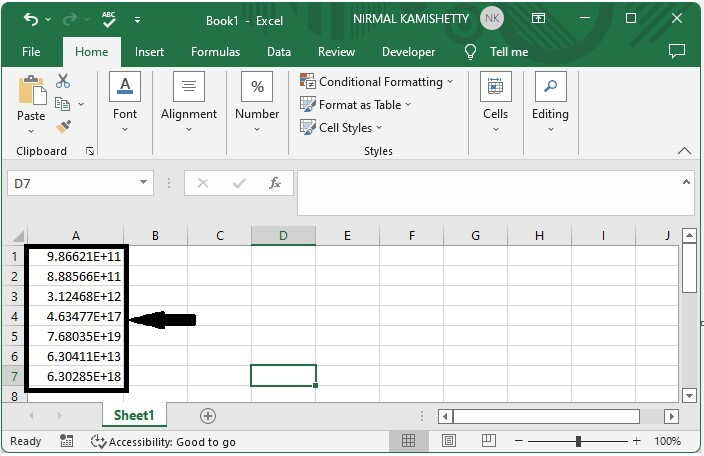Now we click on the cell, enter (‘) before the value, and click enter.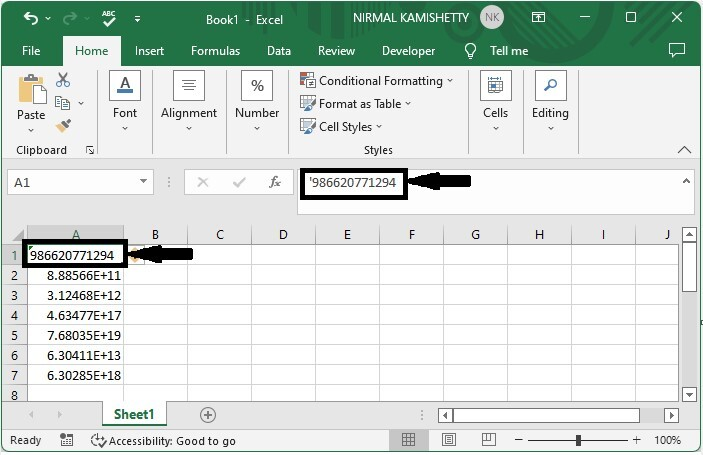### Step 2

Then manually add the single quote before all the values, and our final result will be like the below image.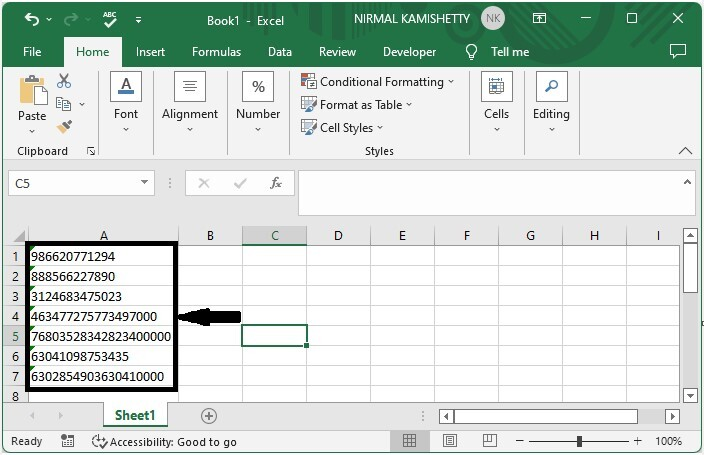## Converting Scientific Notation to Text Using Format

Here, we will use the Format operation to complete the task. Let's take a look at a simple procedure for converting scientific notation to text in Excel.

### Step 1

Let us consider the same data that we used in the above example.

Now select the values, then right-click and select format cells.

Then, in the pop-up window, click "custom," type "0," and then click "OK."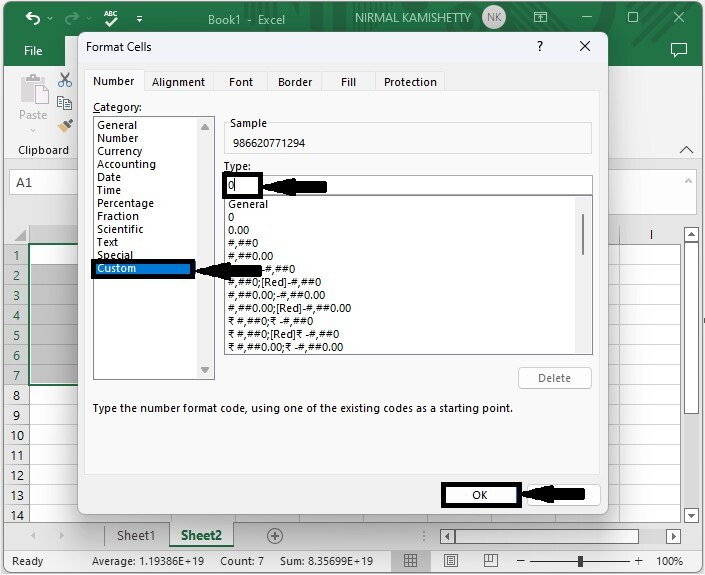## Convert Scientific Notation to Text Using TRIM

Here, we will first use the TRIM formula to get any one of the results, then use the auto-fill handle to get all the results. Let's take a look at a simple procedure for converting scientific notation to text in Excel using trim.

### Step 1

Let us consider the same data that we used in the above example.

Then, in the empty cell, enter the formula =TRIM(A1) and press Enter to obtain our first result.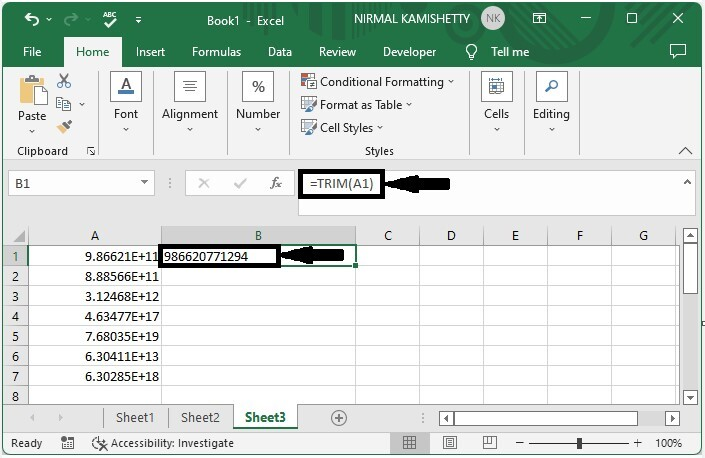### Step 2

To get all the results, drag down from the first result, and our final result will be similar to the below image.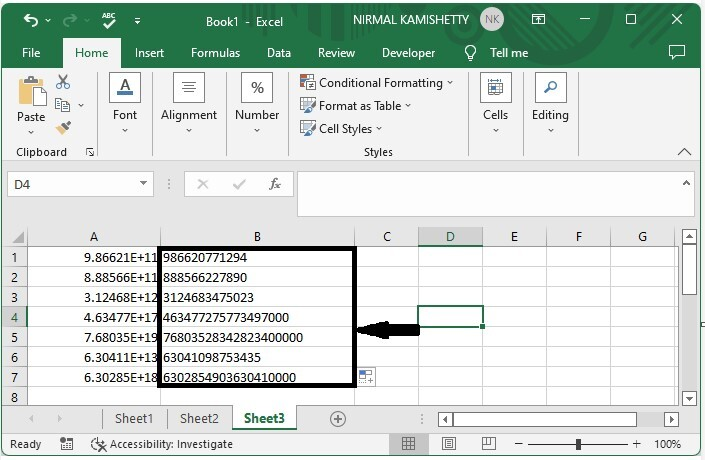## Conclusion

In this tutorial, we used simple examples to demonstrate how you can convert scientific notation to number or text in Excel.

Updated on: 06-Mar-2023

350 Views